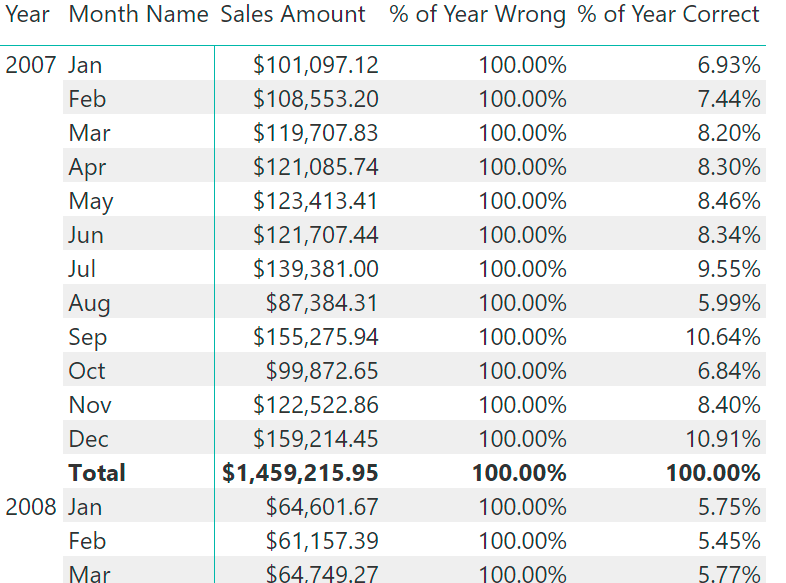A recurring issue for Power BI users writing DAX code is that column filter removal might have an unexpected behavior when the Sort by Column is active on the column. I covered this topic in an article related to RANKX, but it’s not easy to find this problem there, so I think it’s a good idea having a dedicated blog post for that.

Here is the thing: imagine you have this formula:

```% of Year Wrong = DIVIDE (
[Sales Amount],
CALCULATE (
[Sales Amount],
ALL ( 'Date'[Month Name] )
)
)
```

This produces the wrong calculation of the percentage of the year in the matrix below.The reason is that the Month Name column has the property “Sort By Column” set to Month – which is the month number. Otherwise, you would see April as the first month of the year.
This is not a problem if you use Excel as a client, but in Power BI the query generated by DAX has to include both columns in the internal SUMMARIZECOLUMNS generated to display the result. For this reason, a measure that has to modify the filter context modifying the filter on the Month Name column has to include also the month number (the Month column) in the ALL function.

This is the right formula:

```% of Year Correct = DIVIDE (
[Sales Amount],
CALCULATE (
[Sales Amount],
ALL ( 'Date'[Month Name], 'Date'[Month] )
)
)
```

The rule of thumb is the following: whenever you remove a filter from a column that has a Sort By Column property set to another column, you should also include that column in the ALL statement – otherwise, your ALL function might not have the desired effect in the calculation.

SUMMARIZECOLUMNSCreate a summary table for the requested totals over set of groups.

`SUMMARIZECOLUMNS ( [<GroupBy_ColumnName> [, [<FilterTable>] [, [<Name>] [, [<Expression>] [, <GroupBy_ColumnName> [, [<FilterTable>] [, [<Name>] [, [<Expression>] [, … ] ] ] ] ] ] ] ] ] )`

ALLCALCULATE modifier

Returns all the rows in a table, or all the values in a column, ignoring any filters that might have been applied.

`ALL ( [<TableNameOrColumnName>] [, <ColumnName> [, <ColumnName> [, … ] ] ] )`